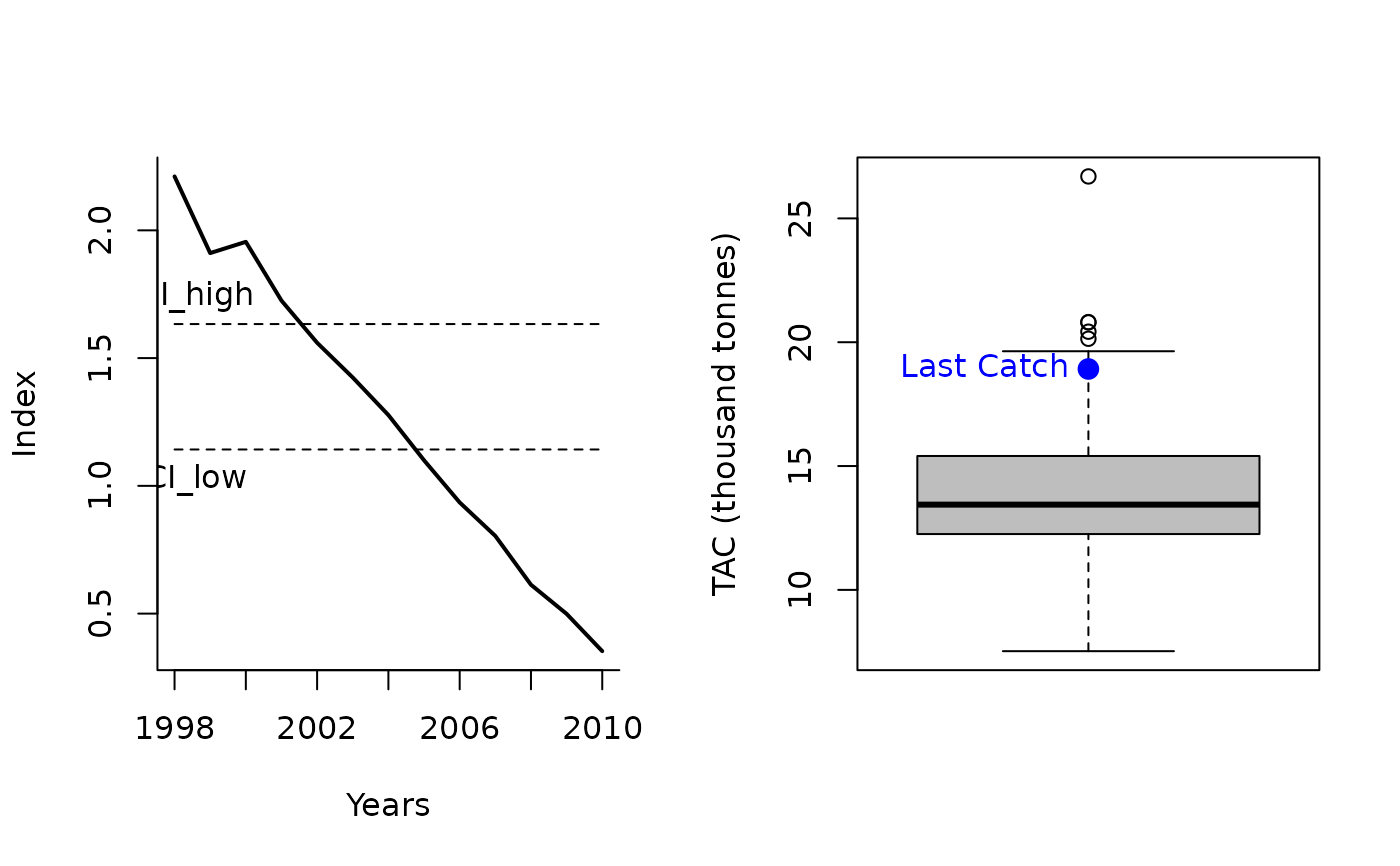The MP adjusts catch based on the value of the index in the current year relative to the time series mean and standard error.

ICI(x, Data, reps = 100, plot = FALSE)

ICI2(x, Data, reps = 100, plot = FALSE)

## Arguments

x

A position in the data object

Data

A data object

reps

The number of stochastic samples of the MP recommendation(s)

plot

Logical. Show the plot?

## Value

An object of class Rec-class with the TAC slot populated with a numeric vector of length reps

## Details

The TAC is calculated as: $$\textrm{TAC}_y=C_{y-1} \alpha$$ where $$C_{y-1}$$ is the catch from the previous year, and $$\alpha$$ is defined as: $$\alpha = \left\{\begin{array}{ll} d & \textrm{if } I < \textrm{CI}_L \\ u & \textrm{if } I > \textrm{CI}_H \\ 1 & \textrm{if } \textrm{CI}_L \leq I \leq \textrm{CI}_H \\ \end{array}\right.$$ where $$I$$ is the index in the most recent year, $$d$$ is 0.75 for ICI and ICI2, $$u$$ is 1.05 and 1.25 forICI and ICI2 respectively, and $$\textrm{CI}_L$$ and $$\textrm{CI}_L$$ are the lower and upper bound of the confidence interval of mean historical index. The confidence interval is calculated using Z-scores described in the Functions section below.

## Functions

• ICI: The catch is reduced by 0.75 if the Z-score of the current year's index is less than -0.44. The catch is increased by 1.05 if the Z-score of the current year's index is greater than 1.96. Otherwise, the catch is held constant.

• ICI2: This method is less precautionary of the two ICI MPs by allowing for a larger increase in TAC and a lower threshold of the index to decrease the TAC. The catch is reduced by 0.75 if the Z-score of the current year's index is less than -1.96. The catch is increased by 1.25 if the Z-score of the current year's index is greater than 1.96. Otherwise, the catch is held constant.

## Required Data

See Data-class for information on the Data object

ICI: Cat, Ind

ICI2: Cat, Ind

## Rendered Equations

See Online Documentation for correctly rendered equations

Other Index methods: GB_slope(), GB_target(), Gcontrol(), Iratio(), Islope1(), Itarget1_MPA(), Itarget1(), ItargetE1()

## Author

Coded by Q. Huynh. Developed by Jardim et al. (2015)

## Examples

ICI(1, Data=MSEtool::Atlantic_mackerel, plot=TRUE)#> TAC (median)
#>     14.14525

ICI2(1, Data=MSEtool::Atlantic_mackerel, plot=TRUE)#> TAC (median)
#>     13.89096